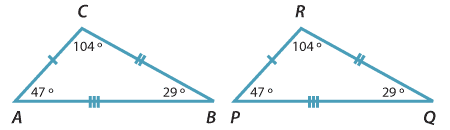### Congruent triangles

When geometric figures are made up entirely of intervals, rather than curves, arguments about congruence can usually be reduced to arguments about congruent triangles. This is one reason why so much attention is given to congruent triangles.

Here are two congruent triangles. The triangle PQR on the right has been formed by a translation of the triangle ABC on the left.The vertices and sides of triangle ABC are matched by the congruence with the vertices and sides of triangle PQR as follows:

 A ↔ P B ↔ Q C ↔ R BC ↔ QR CA ↔ RP AB ↔ PQ

Congruence can be expressed using the symbol $$\equiv$$ for 'is congruent to', and by observing the convention that the two triangles are named with the vertices in matching order:

$$\triangle$$ ABC $$\equiv$$ $$\triangle$$ PQR